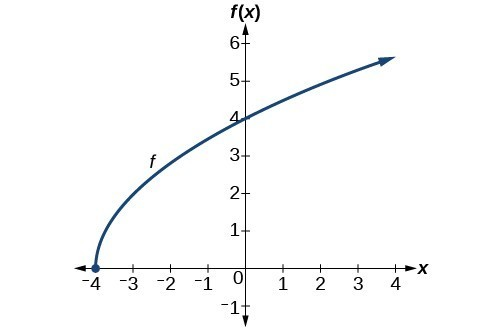## Find domains and ranges of the toolkit functions

We will now return to our set of toolkit functions to determine the domain and range of each.

### How To: Given the formula for a function, determine the domain and range.

1. Exclude from the domain any input values that result in division by zero.
2. Exclude from the domain any input values that have nonreal (or undefined) number outputs.
3. Use the valid input values to determine the range of the output values.
4. Look at the function graph and table values to confirm the actual function behavior.

### Example 8: Finding the Domain and Range Using Toolkit Functions

Find the domain and range of $f\left(x\right)=2{x}^{3}-x$.

### Solution

There are no restrictions on the domain, as any real number may be cubed and then subtracted from the result.

The domain is $\left(-\infty ,\infty \right)$ and the range is also $\left(-\infty ,\infty \right)$.

### Example 9: Finding the Domain and Range

Find the domain and range of $f\left(x\right)=\frac{2}{x+1}$.

### Solution

We cannot evaluate the function at $-1$ because division by zero is undefined. The domain is $\left(-\infty ,-1\right)\cup \left(-1,\infty \right)$. Because the function is never zero, we exclude 0 from the range. The range is $\left(-\infty ,0\right)\cup \left(0,\infty \right)$.

### Example 10: Finding the Domain and Range

Find the domain and range of $f\left(x\right)=2\sqrt{x+4}$.

### Solution

We cannot take the square root of a negative number, so the value inside the radical must be nonnegative.

$x+4\ge 0\text{ when }x\ge -4$

The domain of $f\left(x\right)$ is $\left[-4,\infty \right)$.

We then find the range. We know that $f\left(-4\right)=0$, and the function value increases as $x$ increases without any upper limit. We conclude that the range of $f$ is $\left[0,\infty \right)$.

### Analysis of the Solution

Figure 20 represents the function $f$.Figure 20

### Try It 7

Find the domain and range of $f\left(x\right)=-\sqrt{2-x}$.

Solution